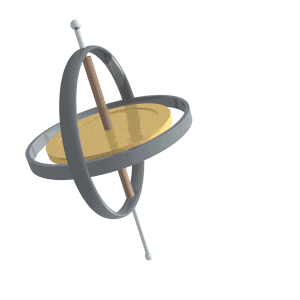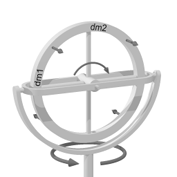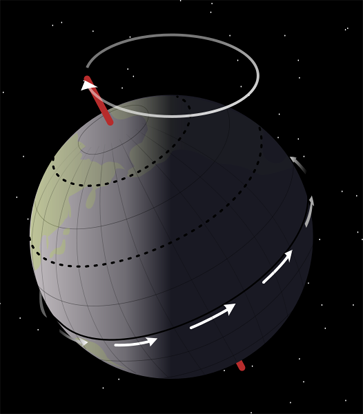PrecessionBy Wikipedia,
the free encyclopedia,

http://en.wikipedia.org/wiki/PrecessionPrecession of a gyroscope

Precession refers to a change in the direction of the axis of a rotating object. More exactly, it can be defined as a change in direction of the rotation axis in which the second Euler angle (nutation) is constant. In physics, there are two types of precession, torque-free and torque-induced, the latter being discussed here in more detail. In certain contexts, "precession" may refer to the precession that the Earth experiences, the effects of this type of precession on astronomical observation, or to the precession of orbital objects.

## Torque-free

Torque-free precession occurs when the axis of rotation differs slightly from an axis about which the object can rotate stably: a maximum or minimum principal axis. Poinsot's construction is an elegant geometrical method for visualizing the torque-free motion of a rotating rigid body. For example, when a plate is thrown, the plate may have some rotation around an axis that is not its axis of symmetry. When an object is not perfectly solid, internal vortices will tend to damp torque-free precession.

Even in a perfectly solid rigid body, torque-free precession will take place when it rotates around an axis in which it is non-symmetrical. This occurs because the angular momentum (L) will have to be constant in the external reference frame (because the hypothesis of torque-free), but the moment of inertia tensor (I) is non-constant in this frame because of the lack of symmetry. Therefore the spin angular velocity vector (ωs) about the spin axis will have to evolve in time so that the matrix product L = I . ωs remains constant.

The torque-free precession rate of an object with an axis of symmetry, such as a disk, spinning about an axis not aligned with that axis of symmetry can be calculated as follows:$\boldsymbol\omega_p = \omega_s \cos(\alpha)((I_s/I_p) - 1)$

where$\boldsymbol\omega_p$ is the precession rate,$\boldsymbol\omega_s$ is the spin rate about the axis of symmetry,$\boldsymbol\alpha$ is the angle between the axis of symmetry and the axis about which it precess,$\boldsymbol I_s$ is the moment of inertia about the axis of symmetry, and$\boldsymbol I_p$ is moment of inertia about either of the other two perpendicular principal axes. They should be the same, due to the symmetry of the disk.

Another type of torque-free precession can occur when there are multiple reference frames at work. For example, the earth is subject to local torque induced precession due to the gravity of the sun and moon acting upon the earth’s axis, but at the same time the solar system is moving around the galactic center. Consequently, an accurate measurement of the earth’s axial reorientation relative to objects outside the frame of the moving galaxy (such as distant quasars commonly used as precession measurement reference points) must account for a minor amount of non-local torque-free precession, due to the solar system’s motion.

## Torque-induced

Torque-induced precession (gyroscopic precession) is the phenomenon in which the axis of a spinning object (e.g. a part of a gyroscope) "wobbles" when a torque is applied to it. The phenomenon is commonly seen in a spinning toy top, but all rotating objects can undergo precession. If the speed of the rotation and the magnitude of the torque are constant the axis will describe a cone, its movement at any instant being at right angles to the direction of the torque. In the case of a toy top, if the axis is not perfectly vertical the torque is applied by the force of gravity tending to tip it over.The device depicted on the right here is gimbal mounted. From inside to outside there are three axes of rotation: the hub of the wheel, the gimbal axis and the vertical pivot.

To distinguish between the two horizontal axes, rotation around the wheel hub will be called 'spinning', and rotation around the gimbal axis will be called 'pitching.' Rotation around the vertical pivot axis is called 'rotation'.

First, imagine that the entire device is rotating around the (vertical) pivot axis. Then, spinning of the wheel (around the wheelhub) is added. Imagine the gimbal axis to be locked, so that the wheel cannot pitch. The gimbal axis has sensors, that measure whether there is a torque around the gimbal axis.

In the picture, a section of the wheel has been named 'dm1'. At the depicted moment in time, section dm1 is at the perimeter of the rotating motion around the (vertical) pivot axis. Section dm1 therefore has a lot of angular rotating velocity with respect to the rotation around the pivot axis, and as dm1 is forced closer to the pivot axis of the rotation (by the wheel spinning further), due to the Coriolis effect dm1 tends to move in the direction of the top-left arrow in the diagram (shown at 45) in the direction of rotation around the pivot axis. Section dm2 of the wheel starts out at the vertical pivot axis, and thus initially has zero angular rotating velocity with respect to the rotation around the pivot axis, before the wheel spins further. A force (again, a Coriolis force) would be required to increase section dm2's velocity up to the angular rotating velocity at the perimeter of the rotating motion around the pivot axis. If that force is not provided, then section dm2's inertia will make it move in the direction of the top-right arrow. Note that both arrows point in the same direction.

The same reasoning applies for the bottom half of the wheel, but there the arrows point in the opposite direction to that of the top arrows. Combined over the entire wheel, there is a torque around the gimbal axis when some spinning is added to rotation around a vertical axis.

It is important to note that the torque around the gimbal axis arises without any delay; the response is instantaneous.

In the discussion above, the setup was kept unchanging by preventing pitching around the gimbal axis. In the case of a spinning toy top, when the spinning top starts tilting, gravity exerts a torque. However, instead of rolling over, the spinning top just pitches a little. This pitching motion reorients the spinning top with respect to the torque that is being exerted. The result is that the torque exerted by gravity - via the pitching motion - elicits gyroscopic precession (which in turn yields a counter torque against the gravity torque) rather than causing the spinning top to fall to its side.

Precession or gyroscopic considerations have an effect on bicycle performance at high speed. Precession is also the mechanism behind gyrocompasses.

Gyroscopic precession also plays a large role in the flight controls on helicopters. Since the driving force behind helicopters is the rotor disk (which rotates), gyroscopic precession comes into play. If the rotor disk is to be tilted forward (to gain forward velocity), its rotation requires that the downward net force on the blade be applied roughly 90 degrees (depending on blade configuration) before, or when the blade is to one side of the pilot and rotating forward.

To ensure the pilot's inputs are correct, the aircraft has corrective linkages which vary the blade pitch in advance of the blade's position relative to the swashplate. Although the swashplate moves in the intuitively correct direction, the blade pitch links are arranged to transmit the pitch in advance of the blade's position.

### Classical (Newtonian)Precessional movement.

The Earth goes through one complete precession cycle in a period of approximately 25,800 years, during which the positions of stars as measured in the equatorial coordinate system will slowly change; the change is actually due to the change of the coordinates. Over this cycle the Earth's north axial pole moves from where it is now, within 1° of Polaris, in a circle around the ecliptic pole, with an angular radius of about 23.5 degrees (or approximately 23 degrees 27 arcminutes ). The shift is 1 degree in 72 years, where the angle is taken from the observer, not from the center of the circle.

Discovery of the precession of the equinoxes is generally attributed to the ancient Greek astronomer Hipparchus (ca. 150 B.C.), though the difference between the sidereal and tropical years (the precession equivalent) was known to Aristarchus of Samos much earlier (ca. 280 B.C.). It was later explained by Newtonian physics. The Earth has a nonspherical shape, being oblate spheroid, bulging outward at the equator. The gravitational tidal forces of the Moon and Sun apply torque as they attempt to pull the equatorial bulge into the plane of the ecliptic. The portion of the precession due to the combined action of the Sun and the Moon is called lunisolar precession.

Revolution of a planet in its orbit around the Sun is also a form of rotary motion. (In this case, the combined system of Earth and Sun is rotating.) So the axis of a planet's orbital plane will also precess over time.

The major axis of each planet's elliptical orbit also precesses within its orbital plane, partly in response to perturbations in the form of the changing gravitational forces exerted by other planets. This is called perihelion precession or apsidal precession (see apsis). Discrepancies between the observed perihelion precession rate of the planet Mercury and that predicted by classical mechanics were prominent among the forms of experimental evidence leading to the acceptance of Einstein's Theory of Relativity, which accurately predicted the anomalies.

These periodic changes of Earth's orbital parameters, combined with the precession of the equinoxes and of the inclination of the Earth's axis on its orbit, are an important part of the astronomical theory of ice ages. For precession of the lunar orbit see lunar precession.

A phenomenon analogous to apsidal precession is nodal precession (see orbital node), which affects the orientation of the orbital plane.

## External links

Text from Wikipedia is available under the Creative Commons Attribution/Share-Alike License; additional terms may apply.

Published - July 2009

Please see some ads intermixed with other content from this site:Copyright 2004-2021 © by Airports-Worldwide.com, Vyshenskoho st. 36, Lviv 79010, Ukraine
Legal Disclaimer